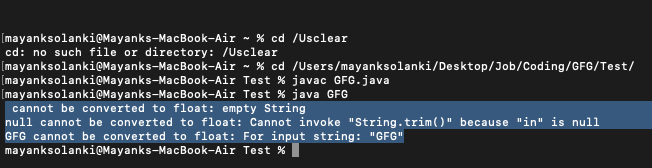Related Articles

# Java Program to Convert String to Float Value

• Last Updated : 05 Aug, 2021

Given a string “str” in Java, the task is to convert this string to float type.

Methods:

This can be done by two methods as listed below:

1. Using parseFloat() Method
2. Using valueOf() Method

Let us discuss above two ways of implementing the methods to get a fair understanding of the conversion of string to float values.

Method 1: Using parseFloat()

The parseFloat() method in Float Class is a built-in method in Java that returns a new float initialized to the value represented by the specified String, as done by the valueOf method of class Float.

Syntax:

`public static float parseFloat(String s) ;`

Parameters: It accepts a single mandatory parameter s which specifies the string to be parsed.

Return type: It returns e float value represented by the string argument.

Exceptions:

• NullPointerException: When the string parsed is null
• NumberFormatException: When the string parsed does not contain a parsable float

Example 1

## Java

 `// Java Program to Convert String to Float Value by``// Implementing parseFloat() method of Float class` `// Importing input output classes``import` `java.io.*;` `// Main class``class` `GFG {` `    ``// Main driver method``    ``public` `static` `void` `main(String[] args)``    ``{``        ``// Input string``        ``String str = ``"100"``;` `        ``// Returning the float value``        ``// represented by the string argument``        ``float` `val = Float.parseFloat(str);` `        ``// Prints the float value of the string``        ``System.out.println(``"String is converted to float "``                           ``+ val);``    ``}``}`
Output
`String is converted to float 100.0`

Example 2: To show unsuccessful conversion

## Java

 `// Java Program to Convert String to Float Value by``// Implementing parseFloat() method of Float class``// Where Exception Message is thrown` `// Importing input output classes``import` `java.io.*;` `// Main class``class` `GFG {` `   ``// Method 1``   ``// To convert string to float``   ``public` `static` `void` `convertStringToFloat(String str) {` `      ``float` `floatValue;` `      ``// Try block to check for exceptions``      ``try` `{` `         ``// Convert string to float``         ``// using parseFloat() method``         ``floatValue = Float.parseFloat(str);` `         ``// Print the expected float value``         ``System.out.println(``            ``str``            ``+ ``" after converting into float = "``            ``+ floatValue);``      ``}` `      ``// Catch block to handle the exception``      ``catch` `(Exception e) {` `         ``// Print the exception message``         ``// using getMessage() method``         ``System.out.println(``            ``str``            ``+ ``" cannot be converted to float: "``            ``+ e.getMessage());``      ``}``   ``}` `   ``// Method 2``   ``// Main driver code``   ``public` `static` `void` `main(String[] args) {` `      ``// Declaring and initializing custom strings values``      ``String str1 = ``""``;``      ``String str2 = ``null``;``      ``String str3 = ``"GFG"``;` `      ``// Convert string to float``      ``// using parseFloat() method``      ``convertStringToFloat(str1);``      ``convertStringToFloat(str2);``      ``convertStringToFloat(str3);``   ``}``}`

Output:Method 2: Using valueof() method

The valueOf() method of the Float class converts data from its internal form into human-readable form. The valueOf() method converts data from its internal form into a human-readable form. It is a static method that is overloaded within a string for all of Java’s built-in types so that each type can be converted properly into a string.

It is called when a string representation of some other type data is needed-for example during concatenation operation.you can call this method with any data type and get a reasonable String representation valueOf() returns java.lang.Integer, which is the object representative of the integer Few forms of valueOf()

Syntax:

`Float.valueOf(str);`

Returns:

• It returns string representation of given value
• valueOf(iNum); // Returns the string representation of int iNum.
• String.valueOf(sta); // Returns the string representation of the boolean argument.
• String.valueOf(fNum); // Returns the string representation of the float fnum.
• String.valueOf(data, 0, 15); // Returns the string representation of a specific subarray of the chararray argument.
• String.valueOf(data, 0, 5); // Returns the string of charArray 0 to 5
• String.valueOf(data, 7, 9); // Returns the string of charArray starting index 7 and total count from 7 is 9.

Example

## Java

 `// Java Program to Convert String to Float Value``// Using valuesOf() method` `// Importing input output classes``import` `java.io.*;` `// Main class``class` `GFG {` `    ``// Method 1``    ``// To convert String to Float``    ``public` `static` `float` `convertStringToFloat(String str)``    ``{``        ``// Convert string to float``        ``// using valueOf() method``        ``return` `Float.valueOf(str);``    ``}` `    ``// Method 2``    ``// Main driver method``    ``public` `static` `void` `main(String[] args)``    ``{` `        ``// Custom input string value``        ``String stringValue = ``"1.0"``;` `        ``// Expected float value``        ``float` `floatValue;` `        ``// Converting string to float``        ``floatValue = convertStringToFloat(stringValue);` `        ``// Printing  the expected float value``        ``System.out.println(``            ``stringValue + ``" after converting into float = "``            ``+ floatValue);``    ``}``}`
Output
`1.0 after converting into float = 1.0`

Attention reader! Don’t stop learning now. Get hold of all the important Java Foundation and Collections concepts with the Fundamentals of Java and Java Collections Course at a student-friendly price and become industry ready. To complete your preparation from learning a language to DS Algo and many more,  please refer Complete Interview Preparation Course.

My Personal Notes arrow_drop_up